Related Articles

# Matplotlib Tutorial

• Last Updated : 07 Jul, 2021

Matplotlib is easy to use and an amazing visualizing library in Python. It is built on NumPy arrays and designed to work with the broader SciPy stack and consists of several plots like line, bar, scatter, histogram, etc.

In this article, we will learn about Python plotting with Matplotlib from basics to advance with the help of a huge dataset containing information about different types of plots and their customizations.

Table Of Content

Recent Articles on Matplotlib !!!

## Getting Started

Before we start learning about Matplotlib we first have to set up the environment and will also see how to use Matplotlib with Jupyter Notebook:

After learning about the environment setup and how to use Matplotlib with Jupyter let’s create a simple plot. We will be plotting two lists containing the X, Y coordinates for the plot.

Example:

## Python3

 `import` `matplotlib.pyplot as plt``   ``# initializing the data``   ``x ``=` `[``10``, ``20``, ``30``, ``40``]``   ``y ``=` `[``20``, ``30``, ``40``, ``50``]``   ``# plotting the data``   ``plt.plot(x, y)``   ``# Adding the title``   ``plt.title(``"Simple Plot"``)``   ``# Adding the labels``   ``plt.ylabel(``"y-axis"``)``   ``plt.xlabel(``"x-axis"``)``   ``plt.show()`

Output: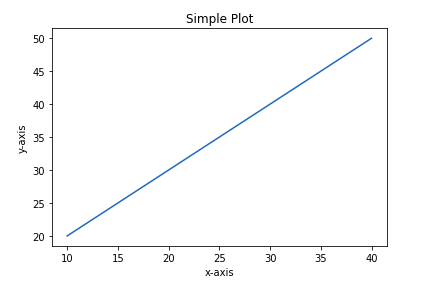In the above example, the elements of X and Y provides the coordinates for the x axis and y axis and a straight line is plotted against those coordinates. For a detailed introduction to Matplotlib and to see how basic charts are plotted refer to the below article.

In the above article, you might have seen Pyplot was imported in code and must have wondered what is Pyplot. Don’t worry we will discuss the Pyplot in the next section.

## Pyplot

Pyplot is a Matplotlib module that provides a MATLAB like interface. Pyplot provides functions that interact with the figure i.e. creates a figure, decorates the plot with labels, creates plotting area in a figure.

Syntax:

matplotlib.pyplot.plot(*args, scalex=True, scaley=True, data=None, **kwargs)

Example:

## Python3

 `# Python program to show pyplot module``   ``import` `matplotlib.pyplot as plt ``   ``plt.plot([``1``, ``2``, ``3``, ``4``], [``1``, ``4``, ``9``, ``16``]) ``   ``plt.axis([``0``, ``6``, ``0``, ``20``]) ``   ``plt.show()`

Output: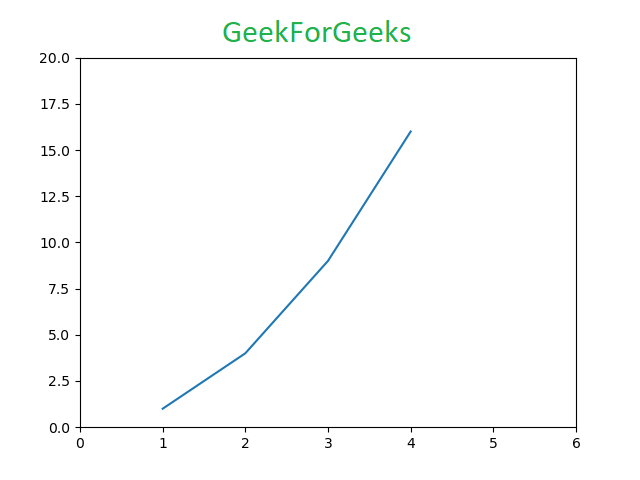Refer to the below articles to get detailed information about Pyplot and functions associated with this class.

>>> More Functions on Pyplot class

Matplotlib take care of the creation of inbuilt defaults like Figure and Axes. Don’t worry about these terms we will study about them in detail in the below section but let’s take a brief about these terms.

• Figure: This class is the top-level container for all the plots means it is the overall window or page on which everything is drawn. A figure object can be considered as a box-like container that can hold one or more axes.
• Axes: This class is the most basic and flexible component for creating sub-plots. You might confuse axes as the plural of axis but it is an individual plot or graph. A given figure may contain many axes but a given axes can only be in one figure.

## Figure class

Figure class is the top-level container that contains one or more axes. It is the overall window or page on which everything is drawn.

Syntax:

class matplotlib.figure.Figure(figsize=None, dpi=None, facecolor=None, edgecolor=None, linewidth=0.0, frameon=None, subplotpars=None, tight_layout=None, constrained_layout=None)

Example 1:

## Python3

 `# Python program to show pyplot module``   ``import` `matplotlib.pyplot as plt ``   ``from` `matplotlib.figure ``import` `Figure ``   ``# Creating a new figure with width = 5 inches``   ``# and height = 4 inches``   ``fig ``=` `plt.figure(figsize ``=``(``5``, ``4``)) ``   ``# Creating a new axes for the figure``   ``ax ``=` `fig.add_axes([``1``, ``1``, ``1``, ``1``]) ``   ``# Adding the data to be plotted``   ``ax.plot([``2``, ``3``, ``4``, ``5``, ``5``, ``6``, ``6``], [``5``, ``7``, ``1``, ``3``, ``4``, ``6` `,``8``])``   ``plt.show()`

Output: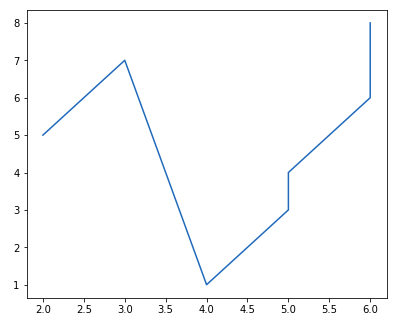Example 2: Creating multiple plots

## Python3

 `# Python program to show pyplot module``   ``import` `matplotlib.pyplot as plt ``   ``from` `matplotlib.figure ``import` `Figure ``   ``# Creating a new figure with width = 5 inches``   ``# and height = 4 inches``   ``fig ``=` `plt.figure(figsize ``=``(``5``, ``4``)) ``   ``# Creating first axes for the figure``   ``ax1 ``=` `fig.add_axes([``1``, ``1``, ``1``, ``1``]) ``   ``# Creating second axes for the figure``   ``ax2 ``=` `fig.add_axes([``1``, ``0.5``, ``0.5``, ``0.5``])``   ``# Adding the data to be plotted``   ``ax1.plot([``2``, ``3``, ``4``, ``5``, ``5``, ``6``, ``6``], [``5``, ``7``, ``1``, ``3``, ``4``, ``6` `,``8``])``   ``ax2.plot([``1``, ``2``, ``3``, ``4``, ``5``], [``2``, ``3``, ``4``, ``5``, ``6``])``   ``plt.show()`

Output: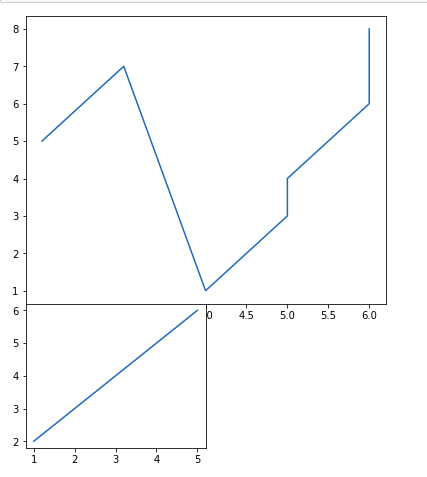Refer to the below articles to get detailed information about the Figure class and functions associated with it.

>>> More Functions in Figure Class

## Axes Class

Axes class is the most basic and flexible unit for creating sub-plots. A given figure may contain many axes, but a given axes can only be present in one figure. The axes() function creates the axes object. Let’s see the below example.

Syntax:

matplotlib.pyplot.axis(*args, emit=True, **kwargs)

Example 1:

## Python3

 `# Python program to show pyplot module``   ``import` `matplotlib.pyplot as plt ``   ``from` `matplotlib.figure ``import` `Figure ``   ``# Creating the axes object with argument as ``   ``# [left, bottom, width, height]``   ``ax ``=` `plt.axes([``1``, ``1``, ``1``, ``1``])`

Output: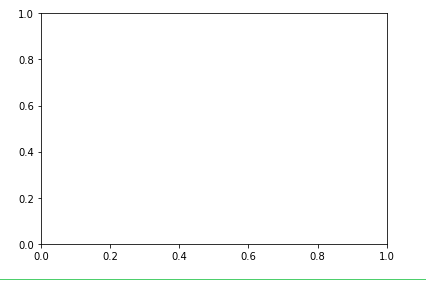Example 2:

## Python3

 `# Python program to show pyplot module``   ``import` `matplotlib.pyplot as plt ``   ``from` `matplotlib.figure ``import` `Figure ``   ``fig ``=` `plt.figure(figsize ``=` `(``5``, ``4``))``   ``# Adding the axes to the figure``   ``ax ``=` `fig.add_axes([``1``, ``1``, ``1``, ``1``])``   ``# plotting 1st dataset to the figure``   ``ax1 ``=` `ax.plot([``1``, ``2``, ``3``, ``4``], [``1``, ``2``, ``3``, ``4``])``   ``# plotting 2nd dataset to the figure``   ``ax2 ``=` `ax.plot([``1``, ``2``, ``3``, ``4``], [``2``, ``3``, ``4``, ``5``])``   ``plt.show()`

Output: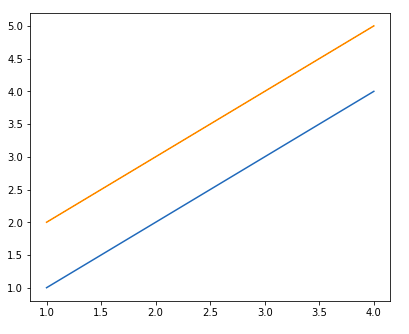Refer to the below articles to get detailed information about the axes class and functions associated with it.

>>> More Functions on Axes Class

## Setting Limits and Tick labels

You might have seen that Matplotlib automatically sets the values and the markers(points) of the x and y axis, however, it is possible to set the limit and markers manually. set_xlim() and set_ylim() functions are used to set the limits of the x-axis and y-axis respectively. Similarly, set_xticklabels() and set_yticklabels() functions are used to set tick labels.

Example:

## Python3

 `# Python program to show pyplot module``   ``import` `matplotlib.pyplot as plt ``   ``from` `matplotlib.figure ``import` `Figure ``   ``x ``=` `[``3``, ``1``, ``3``] ``   ``y ``=` `[``3``, ``2``, ``1``] ``   ``# Creating a new figure with width = 5 inches``   ``# and height = 4 inches``   ``fig ``=` `plt.figure(figsize ``=``(``5``, ``4``)) ``   ``# Creating first axes for the figure``   ``ax ``=` `fig.add_axes([``0.1``, ``0.1``, ``0.8``, ``0.8``]) ``   ``# Adding the data to be plotted``   ``ax.plot(x, y)``   ``ax.set_xlim(``1``, ``2``)``   ``ax.set_xticklabels((``   ``"one"``, ``"two"``, ``"three"``, ``"four"``, ``"five"``, ``"six"``)) ``   ``plt.show()`

Output: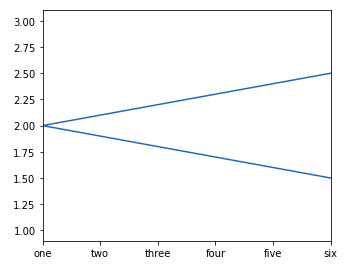## Multiple Plots

Till now you must have got a basic idea about Matplotlib and plotting some simple plots, now what if you want to plot multiple plots in the same figure. This can be done using multiple ways. One way was discussed above using the add_axes() method of the figure class. Let’s see various ways multiple plots can be added with the help of examples.

Method 1: Using the add_axes() method

The add_axes() method figure module of matplotlib library is used to add an axes to the figure.

Syntax:

Example:

## Python3

 `# Python program to show pyplot module``   ``import` `matplotlib.pyplot as plt ``   ``from` `matplotlib.figure ``import` `Figure ``   ``# Creating a new figure with width = 5 inches``   ``# and height = 4 inches``   ``fig ``=` `plt.figure(figsize ``=``(``5``, ``4``)) ``   ``# Creating first axes for the figure``   ``ax1 ``=` `fig.add_axes([``0.1``, ``0.1``, ``0.8``, ``0.8``]) ``   ``# Creating second axes for the figure``   ``ax2 ``=` `fig.add_axes([``0.5``, ``0.5``, ``0.3``, ``0.3``])``   ``# Adding the data to be plotted``   ``ax1.plot([``5``, ``4``, ``3``, ``2``, ``1``], [``2``, ``3``, ``4``, ``5``, ``6``])``   ``ax2.plot([``1``, ``2``, ``3``, ``4``, ``5``], [``2``, ``3``, ``4``, ``5``, ``6``])``   ``plt.show()`

Output: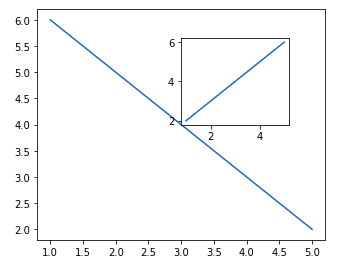The add_axes() method adds the plot in the same figure by creating another axes object.

Method 2: Using subplot() method.

This method adds another plot to the current figure at the specified grid position.

Syntax:

subplot(nrows, ncols, index, **kwargs)

subplot(pos, **kwargs)

subplot(ax)

Example:

## Python3

 `import` `matplotlib.pyplot as plt ``   ``# data to display on plots ``   ``x ``=` `[``3``, ``1``, ``3``] ``   ``y ``=` `[``3``, ``2``, ``1``] ``   ``z ``=` `[``1``, ``3``, ``1``] ``   ``# Creating figure object ``   ``plt.figure() ``   ``# addind first subplot ``   ``plt.subplot(``121``) ``   ``plt.plot(x, y) ``   ``# addding second subplot ``   ``plt.subplot(``122``) ``   ``plt.plot(z, y)`

Output: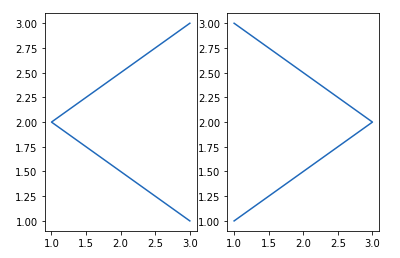Note: Subplot() function have the following disadvantages –

• It does not allow adding multiple subplots at the same time.
• It deletes the preexisting plot of the figure.

Method 3: Using subplots() method

This function is used to create figure and multiple subplots at the same time.

Syntax:

matplotlib.pyplot.subplots(nrows=1, ncols=1, sharex=False, sharey=False, squeeze=True, subplot_kw=None, gridspec_kw=None, **fig_kw)

Example:

## Python3

 `import` `matplotlib.pyplot as plt ``   ``# Creating the figure and subplots``   ``# according the argument passed``   ``fig, axes ``=` `plt.subplots(``1``, ``2``)``   ``# plotting the data in the 1st subplot``   ``axes[``0``].plot([``1``, ``2``, ``3``, ``4``], [``1``, ``2``, ``3``, ``4``])``   ``# plotting the data in the 1st subplot only``   ``axes[``0``].plot([``1``, ``2``, ``3``, ``4``], [``4``, ``3``, ``2``, ``1``])``   ``# plotting the data in the 2nd subplot only``   ``axes[``1``].plot([``1``, ``2``, ``3``, ``4``], [``1``, ``1``, ``1``, ``1``])`

Output: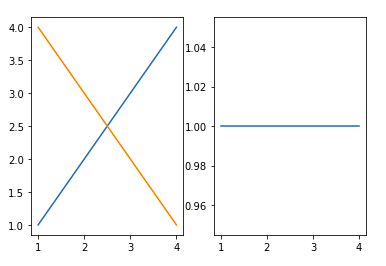Method 4: Using subplot2grid() method

This function give additional flexibility in creating axes object at a specified location inside a grid. It also helps in spanning the axes object across multiple rows or columns. In simpler words, this function is used to create multiple charts within the same figure.

Syntax:

Plt.subplot2grid(shape, location, rowspan, colspan)

Example:

## Python3

 `import` `matplotlib.pyplot as plt ``   ``# data to display on plots ``   ``x ``=` `[``3``, ``1``, ``3``] ``   ``y ``=` `[``3``, ``2``, ``1``] ``   ``z ``=` `[``1``, ``3``, ``1``] ``   ``# adding the subplots``   ``axes1 ``=` `plt.subplot2grid (``   ``(``7``, ``1``), (``0``, ``0``), rowspan ``=` `2``,  colspan ``=` `1``) ``   ``axes2 ``=` `plt.subplot2grid (``   ``(``7``, ``1``), (``2``, ``0``), rowspan ``=` `2``, colspan ``=` `1``) ``   ``axes3 ``=` `plt.subplot2grid (``   ``(``7``, ``1``), (``4``, ``0``), rowspan ``=` `2``, colspan ``=` `1``) ``   ``# plotting the data``   ``axes1.plot(x, y)``   ``axes2.plot(x, z)``   ``axes3.plot(z, y)`

Output: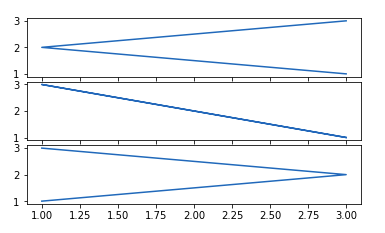Refer to the below articles to get detailed information about subplots

## What is a Legend?

A legend is an area describing the elements of the graph. In simple terms, it reflects the data displayed in the graph’s Y-axis. It generally appears as the box containing a small sample of each color on the graph and a small description of what this data means.

### Creating the Legend

A Legend can be created using the legend() method. The attribute Loc in the legend() is used to specify the location of the legend. The default value of loc is loc=”best” (upper left). The strings ‘upper left’, ‘upper right’, ‘lower left’, ‘lower right’ place the legend at the corresponding corner of the axes/figure.

The attribute bbox_to_anchor=(x, y) of legend() function is used to specify the coordinates of the legend, and the attribute ncol represents the number of columns that the legend has. Its default value is 1.

Syntax:

matplotlib.pyplot.legend([“blue”, “green”], bbox_to_anchor=(0.75, 1.15), ncol=2)

Example:

## Python3

 `import` `matplotlib.pyplot as plt ``   ``# data to display on plots ``   ``x ``=` `[``3``, ``1``, ``3``] ``   ``y ``=` `[``3``, ``2``, ``1``] ``   ``plt.plot(x, y)``   ``plt.plot(y, x)``   ``# Adding the legends``   ``plt.legend([``"blue"``, ``"orange"``])``   ``plt.show()`

Output: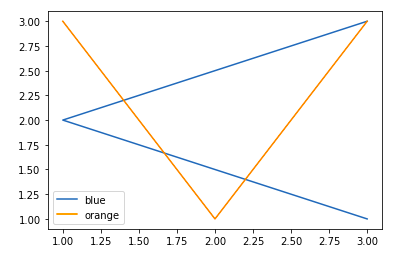Refer to the below articles to get detailed information about the legend –

## Creating Different Types of Plots

### Line Graph

Till now you all must have seen that we are working with only the line charts as they are easy to plot and understand. Line Chart is used to represent a relationship between two data X and Y on a different axis. It is plotted using the pot() function. Let’s see the below example

Example:

## Python3

 `import` `matplotlib.pyplot as plt ``   ``# data to display on plots ``   ``x ``=` `[``3``, ``1``, ``3``] ``   ``y ``=` `[``3``, ``2``, ``1``] ``   ``# This will plot a simple line chart``   ``# with elements of x as x axis and y``   ``# as y axis``   ``plt.plot(x, y)``   ``plt.title(``"Line Chart"``)``   ``# Adding the legends``   ``plt.legend([``"Line"``])``   ``plt.show()`

Output: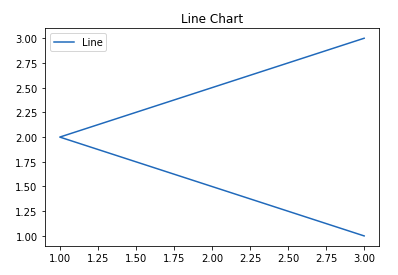Refer to the below article to get detailed information about line chart.

### Bar chart

A bar plot or bar chart is a graph that represents the category of data with rectangular bars with lengths and heights that is proportional to the values which they represent. The bar plots can be plotted horizontally or vertically. A bar chart describes the comparisons between the discrete categories. It can be created using the bar() method.

Syntax:

plt.bar(x, height, width, bottom, align)

Example:

## Python3

 `import` `matplotlib.pyplot as plt ``   ``# data to display on plots ``   ``x ``=` `[``3``, ``1``, ``3``, ``12``, ``2``, ``4``, ``4``] ``   ``y ``=` `[``3``, ``2``, ``1``, ``4``, ``5``, ``6``, ``7``] ``   ``# This will plot a simple bar chart``   ``plt.bar(x, y)``   ``# Title to the plot``   ``plt.title(``"Bar Chart"``)``   ``# Adding the legends``   ``plt.legend([``"bar"``])``   ``plt.show()`

Output: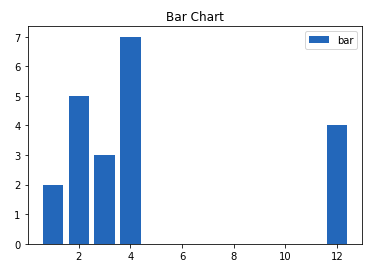Refer to the below articles to get detailed information about Bar charts –

### Histograms

A histogram is basically used to represent data in the form of some groups. It is a type of bar plot where the X-axis represents the bin ranges while the Y-axis gives information about frequency. To create a histogram the first step is to create a bin of the ranges, then distribute the whole range of the values into a series of intervals, and count the values which fall into each of the intervals. Bins are clearly identified as consecutive, non-overlapping intervals of variables. The hist() function is used to compute and create histogram of x.

Syntax:

matplotlib.pyplot.hist(x, bins=None, range=None, density=False, weights=None, cumulative=False, bottom=None, histtype=’bar’, align=’mid’, orientation=’vertical’, rwidth=None, log=False, color=None, label=None, stacked=False, \*, data=None, \*\*kwargs)

Example:

## Python3

 `import` `matplotlib.pyplot as plt ``   ``# data to display on plots ``   ``x ``=` `[``1``, ``2``, ``3``, ``4``, ``5``, ``6``, ``7``, ``4``] ``   ``# This will plot a simple histogram``   ``plt.hist(x, bins ``=` `[``1``, ``2``, ``3``, ``4``, ``5``, ``6``, ``7``])``   ``# Title to the plot``   ``plt.title(``"Histogram"``)``   ``# Adding the legends``   ``plt.legend([``"bar"``])``   ``plt.show()`

Output: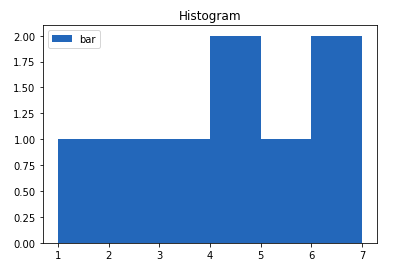Refer to the below articles to get detailed information about histograms.

### Scatter Plot

Scatter plots are used to observe relationship between variables and uses dots to represent the relationship between them. The scatter() method in the matplotlib library is used to draw a scatter plot.

Syntax:

matplotlib.pyplot.scatter(x_axis_data, y_axis_data, s=None, c=None, marker=None, cmap=None, vmin=None, vmax=None, alpha=None, linewidths=None, edgecolors=None)

Example:

## Python3

 `import` `matplotlib.pyplot as plt ``   ``# data to display on plots ``   ``x ``=` `[``3``, ``1``, ``3``, ``12``, ``2``, ``4``, ``4``]``   ``y ``=` `[``3``, ``2``, ``1``, ``4``, ``5``, ``6``, ``7``]``   ``# This will plot a simple scatter chart``   ``plt.scatter(x, y)``   ``# Adding legend to the plot``   ``plt.legend(``"A"``)``   ``# Title to the plot``   ``plt.title(``"Scatter chart"``)``   ``plt.show()`

Output: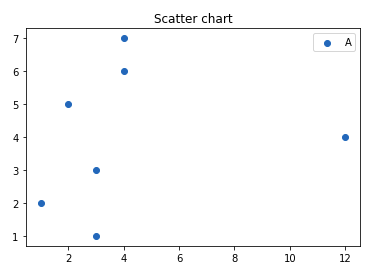Refer to the below articles to get detailed information about the scatter plot.

### Pie Chart

A Pie Chart is a circular statistical plot that can display only one series of data. The area of the chart is the total percentage of the given data. The area of slices of the pie represents the percentage of the parts of the data. The slices of pie are called wedges. The area of the wedge is determined by the length of the arc of the wedge. It can be created using the pie() method.

Syntax:

matplotlib.pyplot.pie(data, explode=None, labels=None, colors=None, autopct=None, shadow=False)

Example:

## Python3

 `import` `matplotlib.pyplot as plt ``   ``# data to display on plots ``   ``x ``=` `[``1``, ``2``, ``3``, ``4``] ``   ``# this will explode the 1st wedge``   ``# i.e. will separate the 1st wedge``   ``# from the chart``   ``e  ``=``(``0.1``, ``0``, ``0``, ``0``)``   ``# This will plot a simple pie chart``   ``plt.pie(x, explode ``=` `e)``   ``# Title to the plot``   ``plt.title(``"Pie chart"``)``   ``plt.show()`

Output: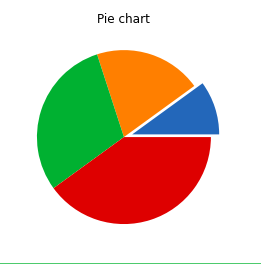Refer to the below articles to get detailed information about pie charts.

### 3D Plots

Matplotlib was introduced keeping in mind, only two-dimensional plotting. But at the time when the release of 1.0 occurred, the 3D utilities were developed upon the 2D and thus, we have a 3D implementation of data available today.

Example:

## Python3

 `import` `matplotlib.pyplot as plt ``   ``# Creating the figure object``   ``fig ``=` `plt.figure()``   ``# keeping the projection = 3d``   ``# ctreates the 3d plot``   ``ax ``=` `plt.axes(projection ``=` `'3d'``)`

Output: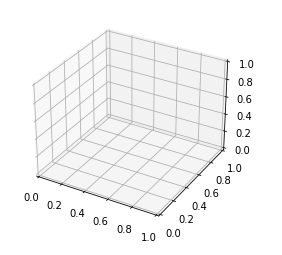The above code lets the creation of a 3D plot in Matplotlib. We can create different types of 3D plots like scatter plots, contour plots, surface plots, etc. Let’s create a simple 3D line plot.

Example:

## Python3

 `import` `matplotlib.pyplot as plt ``   ``x ``=` `[``1``, ``2``, ``3``, ``4``, ``5``]``   ``y ``=` `[``1``, ``4``, ``9``, ``16``, ``25``]``   ``z ``=` `[``1``, ``8``, ``27``, ``64``, ``125``]``   ``# Creating the figure object``   ``fig ``=` `plt.figure()``   ``# keeping the projection = 3d``   ``# ctreates the 3d plot``   ``ax ``=` `plt.axes(projection ``=` `'3d'``)``   ``ax.plot3D(z, y, x)`

Output: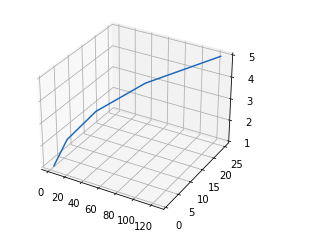Refer to the below articles to get detailed information about 3D plots.

## Working with Images

The image module in matplotlib library is used for working with images in Python. The image module also includes two useful methods which are imread which is used to read images and imshow which is used to display the image.

Example:

## Python3

 `# importing required libraries ``   ``import` `matplotlib.pyplot as plt ``   ``import` `matplotlib.image as img ``   ``# reading the image ``   ``testImage ``=` `img.imread(``'g4g.png'``) ``   ``# displaying the image ``   ``plt.imshow(testImage) `

Output: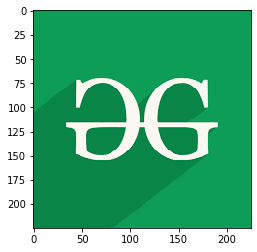Refer to the below articles to get detailed information about working with images using Matplotlib.

## Exercises, Applications, and Projects

Attention geek! Strengthen your foundations with the Python Programming Foundation Course and learn the basics.

To begin with, your interview preparations Enhance your Data Structures concepts with the Python DS Course. And to begin with your Machine Learning Journey, join the Machine Learning – Basic Level Course

My Personal Notes arrow_drop_up